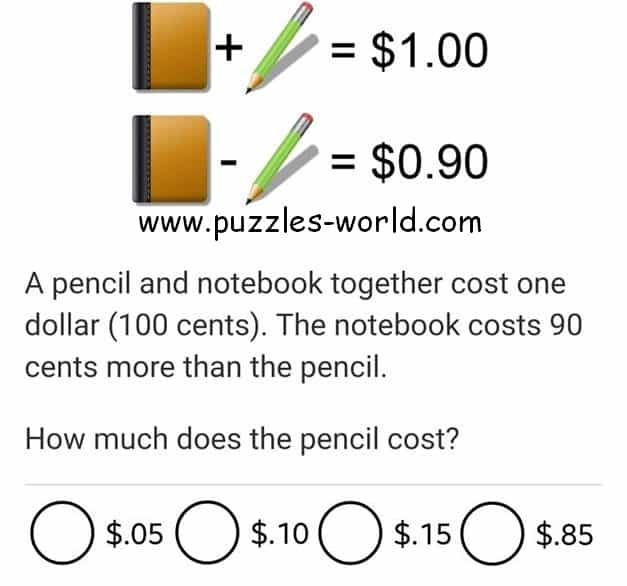# Cost of pencil and notebook puzzleCost of pencil and notebook puzzleA pencil and notebook together cost one dollar (100 cents).
The notebook costs 90 cents more then the pencil.

How much does the pencil cost?

1. \$0.05
2. \$0.10
3. \$0.15
4. \$0.85

#### Click here for Answer

Since the cost of Pencil is \$0.90 less than notebook,
the cost of Notebook is \$0.95 and
the Cost of Pencil is \$0.05